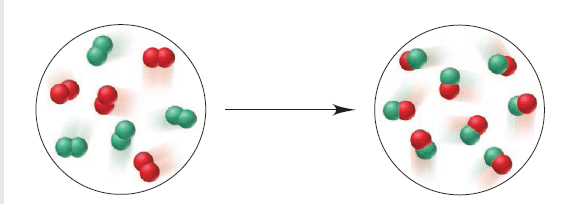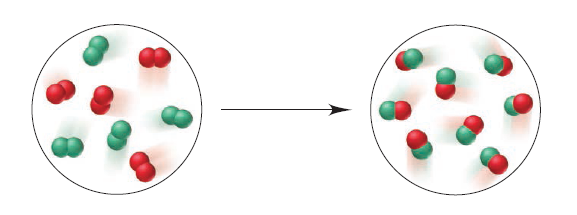# Problem: The scenes below represent a chemical reaction between elements A (red) and B (green):Which best represents the balanced equation for the reaction?(a) 2A + 2B ⟶A2 + B2(b) A2 + B2 ⟶2AB(c) B2 + 2AB ⟶2B2 + A2 (d) 4A2 + 4B2 ⟶8AB

###### FREE Expert Solution

For this problem, we have to find which best represents the balanced equation for the reaction

Counting the red and green molecules on the reactant side:

4A+ 4B2

100% (459 ratings)###### Problem Details

The scenes below represent a chemical reaction between elements A (red) and B (green):

Which best represents the balanced equation for the reaction?

(a) 2A + 2B ⟶A2 + B2
(b) A2 + B2 ⟶2AB
(c) B2 + 2AB ⟶2B2 + A
(d) 4A2 + 4B2 ⟶8ABFrequently Asked Questions

What scientific concept do you need to know in order to solve this problem?

Our tutors have indicated that to solve this problem you will need to apply the Balancing Chemical Equations concept. You can view video lessons to learn Balancing Chemical Equations. Or if you need more Balancing Chemical Equations practice, you can also practice Balancing Chemical Equations practice problems.

What professor is this problem relevant for?

Based on our data, we think this problem is relevant for Professor Shoemaker's class at PSU.

What textbook is this problem found in?

Our data indicates that this problem or a close variation was asked in Chemistry: The Molecular Nature of Matter and Change - Silberberg 8th Edition. You can also practice Chemistry: The Molecular Nature of Matter and Change - Silberberg 8th Edition practice problems.